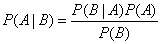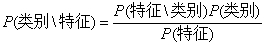出租广告位,需要合作请联系站长

+关注

2020-06(12)

2020-07(30)

2020-08(30)

2020-09(66)

2020-10(79)

## 朴素贝叶斯算法——文本分类（离散型）

P（X）：取值在[0,1]

记作：P（A，B）

记作：P(A|B)``import numpy as np def loadDataSet():    """    加载训练数据, postingList是所有的训练集, 每一个列表代表一条言论, 一共有6条言论 classVec代表每一条言论的类别,     0是正常, 1是有侮辱性 返回 言论和类别    :return:    """    # 步骤的缺失，和正常的比较，此处省略了文本的切割，都是直接弄好的，直接切割成单词用了    postingList=[['my', 'dog', 'has', 'flea', 'problems', 'help', 'please'],                 ['maybe', 'not', 'take', 'him', 'to', 'dog', 'park', 'stupid'],                 ['my', 'dalmation', 'is', 'so', 'cute', 'I', 'love', 'him'],                 ['stop', 'posting', 'stupid', 'worthless', 'garbage'],                 ['mr', 'licks', 'ate', 'my', 'steak', 'how', 'to', 'stop', 'him'],                 ['quit', 'buying', 'worthless', 'dog', 'food', 'stupid']]    classVec = [0,1,0,1,0,1]    return postingList,classVec def createVocabList(dataSet):    """    创建词汇表, 就是把这个文档中所有的单词不重复的放在一个列表里面    :param dataSet:    :return:    """    vocabSet = set([])    for data in dataSet:        # 此处的|表示合并两个集合        vocabSet = vocabSet | set(data)    return list(vocabSet) def setofword(vacablist,inputdata):    """    制作词向量矩阵    将每一个文档转换为词向量, 然后放入矩阵中    :param vocabSet:    :param dataSet:    :return:    """    vectors=[]    # 两个向量相乘，一开始默认都是0    vectors =  * len(vacablist)    for word in inputdata:        if word in vacablist:            vectors[vacablist.index(word)] = 1        else:            print(f'没有{word}这个词')               # print('没有 {} 这个词'.format(word))    return vectors def trainNB1(trainMat,trainCategory):    """    根据上面set集合提取出来，一共有32个特征    trainMat是文档矩阵[]32位0,1，trainCategory是每篇文档类别标签所构成的向量[0,1,0,1,0,1]    """    # 总的训练语句数=文档矩阵的条数6    numTrainDocs = len(trainMat)    # 总的词数 此处为32    numWords = len(trainMat)    # 矩阵的sum是所有元素相加，此处是0+1+0+1+0+1=3    sumOfCate1 = sum(trainCategory)    # 6-3 = 3    sumOfCate0 = len(trainCategory) - sumOfCate1    # 侮辱性词汇的概率 = 3/6 =0.5    pAbusive = sumOfCate1/float(numTrainDocs)    # 创建一个32位的1矩阵，表示初始的p0，为了避免出现零的情况，根据拉普拉斯公式创建1填充的矩阵    p0Num = np.ones(numWords)    # 创建一个32位的1矩阵，表示初始的p1，为了避免出现零的情况，根据拉普拉斯公式创建1填充的矩阵    p1Num = np.ones(numWords)    # 创建一个变量p0Denom 非侮辱性词汇总数，为了避免出现零的情况，根据拉普拉斯公式设置为2.0    p0Denom = 2.0    # 创建一个变量p1Denom 侮辱性词汇总数，为了避免出现零的情况，根据拉普拉斯公式设置为2.0    p1Denom = 2.0    #计算每个词在对应类别中出现的样本数量    for i in range(numTrainDocs):        if trainCategory[i] == 1:            # 最终得到一个侮辱性的32位矩阵            p1Num += trainMat[i]            p1Denom += sum(trainMat[i])        else:            # 最终得到一个非侮辱性的32位矩阵            p0Num += trainMat[i]            p0Denom += sum(trainMat[i])    # log不能直接对矩阵进行操作，np.log    # 词出现的样本数与一个分类样本所有样本数量的比    # 得到侮辱性词汇概率矩阵 （矩阵除以一个浮点数）    # 为了防止下溢出的问题，把矩阵转化成对数的形式，f(x)与ln(f(x))趋势和极值点相同    p1Vect = np.log(p1Num/p1Denom)    # 得到非侮辱性词汇概率矩阵 （矩阵除以一个浮点数）    p0Vect = np.log(p0Num/p0Denom)    return p0Vect,p1Vect,pAbusive # 用得到的概率分类def classfyNB(vec2Classify, p0Vect, p1Vect, pAbusive):    """    参数包括要分类的向量vec2Classify    以及使用函数trainNB1()计算得到的三个概率    """    # 使用numpy数组来计算两个向量相乘的结果，这里的相乘是指对应元素相乘，    # 即先将两个向量中的第一个元素相乘，然后将第二个元素相乘，以此类推    p1 = sum(vec2Classify * p1Vect) + np.log(pAbusive)    p0 = sum(vec2Classify * p0Vect) + np.log(1.0 - pAbusive)    if p1 > p0:        return 1    else:        return 0 #测试代码def testingNB():    print('数字0表示没有侮辱性，数字1表示有侮辱性：')    dataSet, listClasses = loadDataSet()    myVocabList = createVocabList(dataSet)    # print(f'我是词袋长度{len(myVocabList)}')    # print(f'我是词袋{dataSet}')    trainMat = []    for data in dataSet:        trainMat.append(setofword(myVocabList,data))    # print(f'我是矩阵{trainMat}')    p0V,p1V,pAb = trainNB1(trainMat, listClasses)    # print(f'我是概率{p0V,p1V}')    testEntry = ['love','my','dalmation']    thisDOc =setofword(myVocabList,testEntry)    p1 = classfyNB(thisDOc,p0V,p1V,pAb)    print(f'当前词条{testEntry}的结果为：{p1}')    testEntry = ['dog','stupid']    thisDOc =setofword(myVocabList, testEntry)    p2 = classfyNB(thisDOc, p0V, p1V, pAb)    print(f'当前词条{testEntry}的结果为：{p2}') testingNB()``

28 0

pdf(new) 更多>